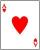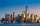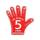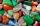Boys and girls

There are eight boys and nine girls in the class. There were six children on the trip from this class. What is the probability that left

a) only boys
b) just two boys

Result

p1 =  0.226 %
p2 =  28.507 %

Solution:Leave us a comment of this math problem and its solution (i.e. if it is still somewhat unclear...):Be the first to comment!To solve this verbal math problem are needed these knowledge from mathematics:

Would you like to compute count of combinations? See also our permutations calculator. See also our variations calculator.

Next similar examples:

1. ClassroomOf the 26 pupils in the classroom, 12 boys and 14 girls, four representatives are picked to the odds of being: a) all the girls b) three girls and one boy c) there will be at least two boys
2. DivisionDivision has 18 members: 10 girls and 6 boys, 2 leaders. How many different patrols can be created, if one patrol is 2 boys, 3 girls and 1 leader?
3. Hearts5 cards are chosen from a standard deck of 52 playing cards (13 hearts) with replacement. What is the probability of choosing 5 hearts in a row?
4. BallsFrom the urn in which are 7 white balls and 17 red, gradually drag 3-times without replacement. What is the probability that pulls balls are in order: red red red?
5. Theorem proveWe want to prove the sentence: If the natural number n is divisible by six, then n is divisible by three. From what assumption we started?
6. MumbaiA job placement agency in Mumbai had to send ten students to five companies two to each. Two of the companies are in Mumbai and others are outside. Two of the students prefer to work in Mumbai while three prefer to work outside. In how many ways assignment
7. Calculation of CNCalculate: ?
8. WordWhat is the probability that a random word composed of chars D, G, O will be the DOG?
9. ValueFind the value of the expression: 6!·10^-3
10. A studentA student is to answer 8 out of 10 questions on the exam. a) find the number n of ways the student can choose 8 out of 10 questions b) find n if the student must answer the first three questions c) How many if he must answer at least 4 of the first 5 que
11. Fish tankA fish tank at a pet store has 8 zebra fish. In how many different ways can George choose 2 zebra fish to buy?
12. A pizzaA pizza place offers 14 different toppings. How many different three topping pizzas can you order?
13. TokensIn the non-transparent bags are red, white, yellow, blue tokens. We 3times pull one tokens and again returned it, write down all possibilities.
14. CardsThe player gets 8 cards of 32. What is the probability that it gets a) all 4 aces b) at least 1 ace
15. One greenIn the container are 45 white and 15 balls. We randomly select 5 balls. What is the probability that it will be a maximum one green?On the menu are 12 kinds of meal. How many ways can we choose four different meals into the daily menu?A book contains 524 pages. If it is known that a person will select any one page between the pages numbered 125 and 384, find the probability of choosing the page numbered 252 or 253.